# Photon Mapping With Color Bleeding

### Description

A program that generates a photon map to render more realistic images than ray tracing and is faster than Monte Carlo Ray Tracing. (written in C++ using the Eigen library)

### Features

• Photon Map generation.
• Partial Russian Roulette to simulate photon emmision.
• Cornell Box to help visualization.
• All three kinds of photons (direct, indirect, shadows).
• BRDF calculations to get more realistic approximation of photons.

### Notes:

• Currently this only works with spheres and planes.
• The actual Russian Roulette method has not been implemented.
• This implementation does not implement caustics.
• Currently the photons have an initial intensity of 1, are summed, averaged, and finally scaled to simulate a good representation of the scene.

### General Algorithm:

#### First Pass: Photon Map

1. For each light, push n photons with intensity 1 out from it in random directions.
2. Trace photon until it collides with an object.
3. Randomly find whether or not the photon is reflected or absorbed.(Normally here you would use Russian Roulette, however I misinterpretted Jensen's explanation and as such did it like this. Russian Roulette is described correctly below).
• Add photon with diffuse of object times its intensity as its new intensity.
• Find shadow photons.
• Take point far along ray.
• Reverse ray, have it start at the point.
• If it intersects and the point isn't the original intersection point, add shadow photon at that point with intesity being the ambient color of the object.
• If object it intersects is the same as the one for the original, done!
• Otherwise, continue from intersection and repeat.
• If photon is absorbed, done!
• If photon is reflected, trace photon with respect to its reflection ray calculated from its incidence ray and the surface normal.
• Repeat.
4. Build KDTree (similar to Jensen's implementation) to store the photons.
• Find largest dimension d of box surrounding all photons.
• Find the median photon with respect to the d value of the point.
• Set the median as the node.
• Build the left subtree with all the photons with its d value less than the median.
• Build the right subtree with all the photons with its d value greater than the median.

#### Second Pass: Rendering

1. Trace rays from camera to scene with respect to the look at point.
2. Set sample square dist (ssd).
3. Find point where ray intersects nearest object.
4. Use Jensen's photon locator KDTree traversing algorithm.
• Check if you are at maximum depth.
• Find distance from point to splitting plane.
• If distance is less than 0, locate photons near left subtree. Then check if squared distance is less than ssd, if it is then search right subtree.
• If distance is greater than 0, locate photons near right subtree. Then check if squared distance is less than ssd, if it is then search left subtree.
• Find squared distance from current photon to point.
• If distance is less than ssd, push photon onto heap. If heap has as many photons as you want, shrink ssd to the distance.
5. Average the intensities of all the photons multiplied by the BRDF calculation, brightening the non shadow photons during which.
6. Divide the average by the area of the sampling sphere.
7. Calculate phong shading of vertex, and add the calculated intesity.

### Actual Russian Roulette:

1. Calculate probability to do a diffuse reflect Pd.
2. Calculate probability to do a specular reflect Ps.
3. Randomly select number from 0 to 1.
4. If number is less than Pd, do a diffuse reflection.
• Put photon on surface it intersects (absorb).
• Bounce photon in random direction with respect to the normal of the surface.
• Repeat.
5. If number is less than Pd plus Ps, do a specular reflection.
• Do exactly what I do, but don't absorb it at the beginning.
• Repeat.
6. Otherwise, just absorb the photon.

### Pictures

#### Initial ray tracing version.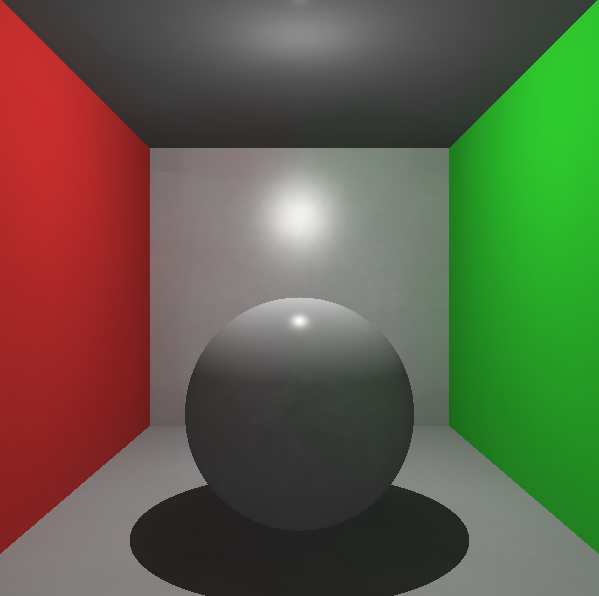Original ray trace versions of my scenes.

#### Photon Map.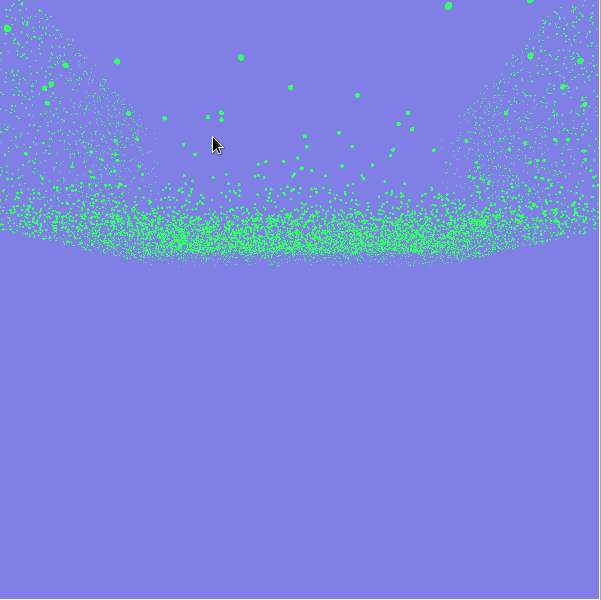Using 20,000 photons, and ray tracing with each acting as a sphere.
Light is directly above the sphere from now on.

#### KDTree Build and Traversing.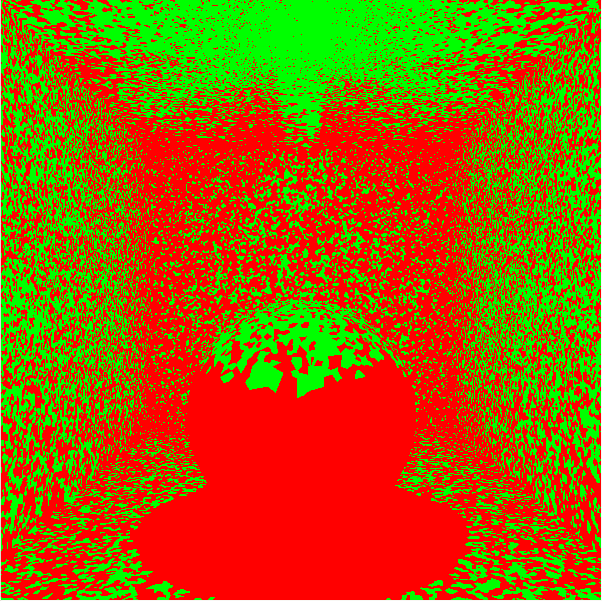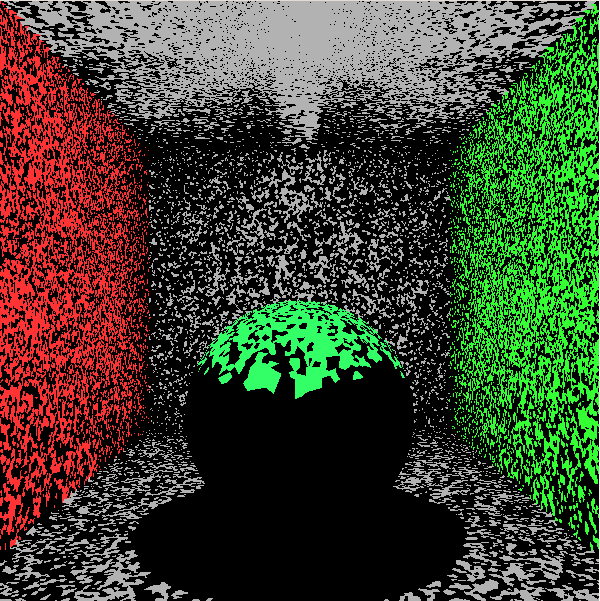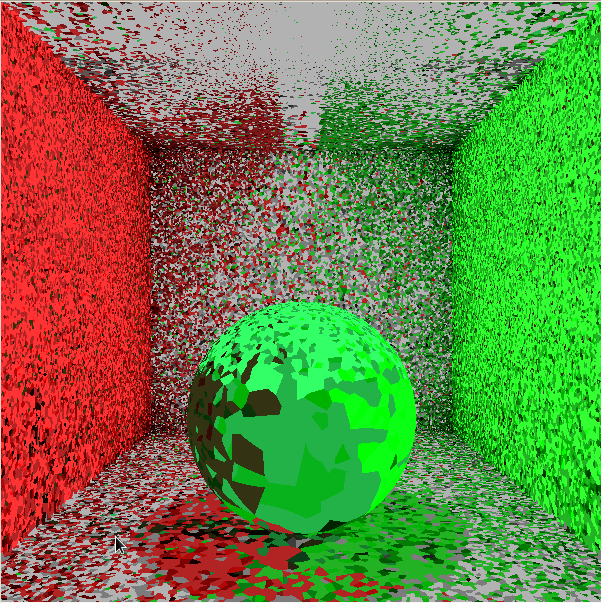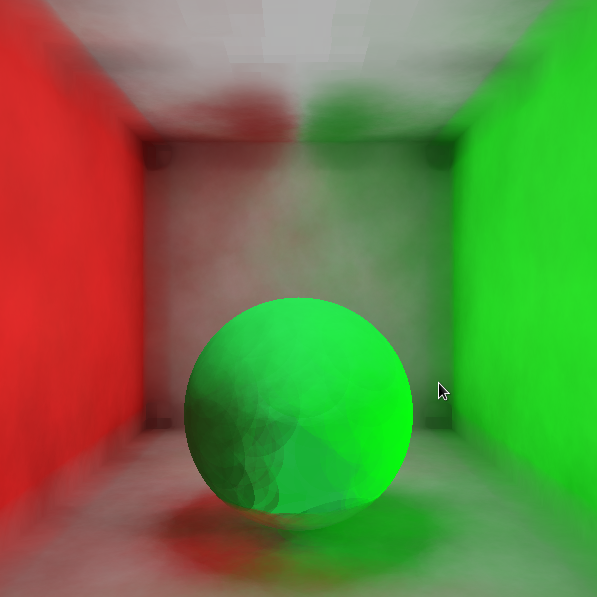Form the ktree and traverse to find direct illumination photons (green) and indirect photons (red).
Color vertices according to DI photons.
Color vertices according to both types of photons.
Adjust sampling to get around 1000 photons, ssd of 0.5, and 100000 photon emmittance.

#### Shadow Photons.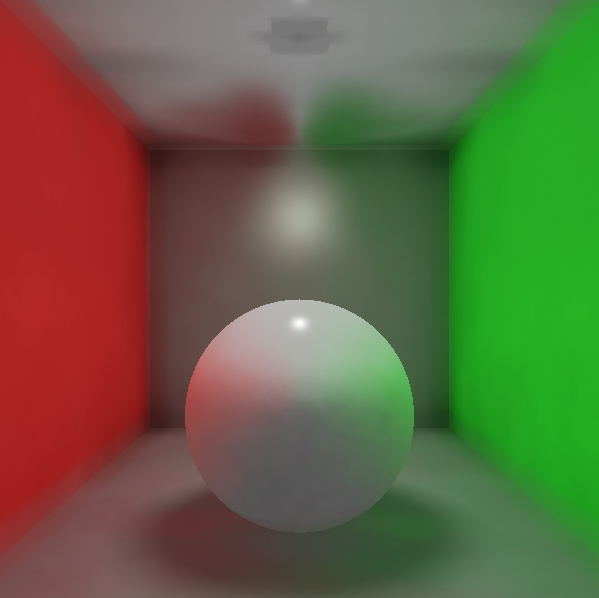No shadow photons on left, exist on right.
Notice the increased shade below sphere and on bottom half of sphere.

#### Scaling.Left image is with emmitting 1 million photons, having overall 3.2 million, sampling for 10,000 photons, ssd of 0.5.
Right image is with emmitting 3 million photons, having overall 7.3 million, sampling for 10,000 photons, ssd of 0.5.
The latter takes approximately 20 seconds to render.

#### BRDF Calculations.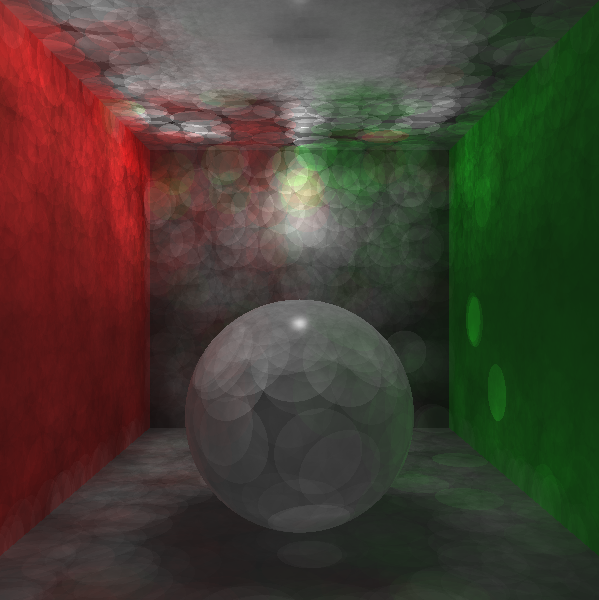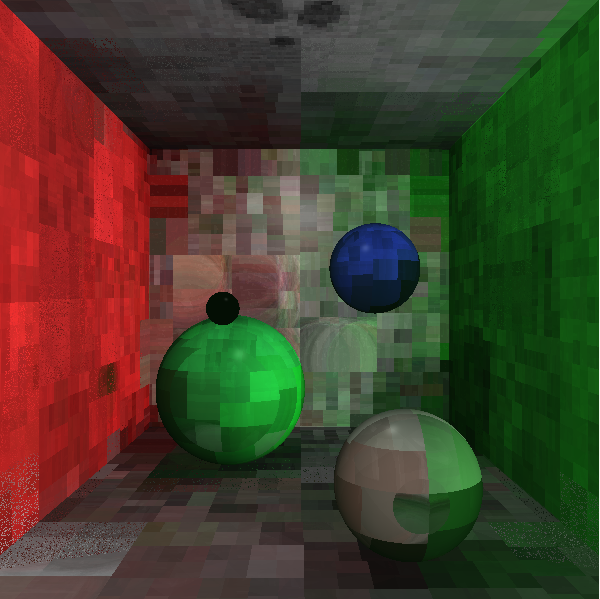Initial changes in intensities with the BRDF calculations and slight modifications in the sampling.

#### Finished.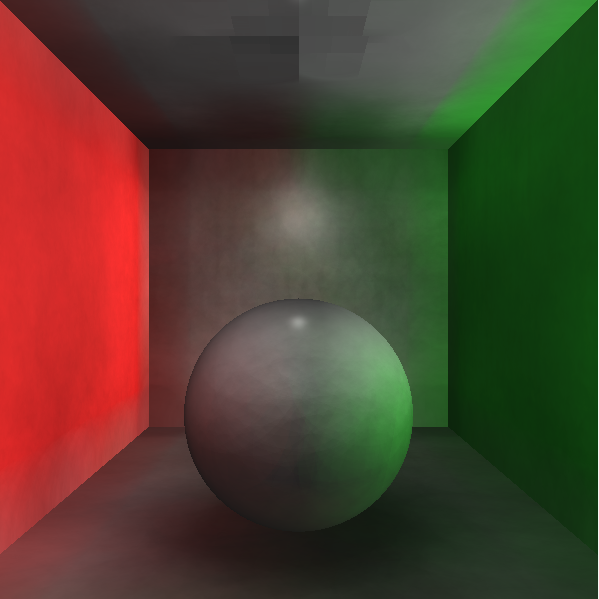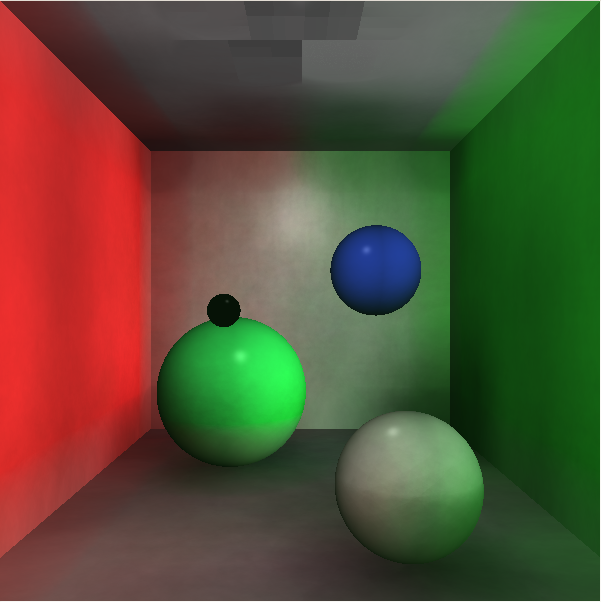Final results
Sampling with 300,000 photons, for 10,000 photons with an ssd of 1.0.
Rendering takes about a minute.

### Sources

• Henrik Wann Jensen, http://graphics.ucsd.edu/~henrik/papers/photon_map/
• Henrik Wann Jensen, http://www.cs.princeton.edu/courses/archive/fall02/cs526/papers/course43sig02.pdf
• Zoe Wood for help and Image.* files.

### Bugs / Unfinished Areas of this Project

• I do not have the actual Russian Roulette implemented.
• Odd grid on top of BRDF renders caused by shadow photons.